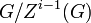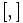# Nilpotent group

Jump to: navigation, search

## Definition

### Equivalent definitions in tabular format

No. Shorthand A group is termed nilpotent if ... A group$G$ is termed nilpotent if ...
1 upper central series its upper central series stabilizes after a finite length at the whole group there is a nonnegative integer$c$ such that$Z^c(G) = G$. Here, we define$Z^i(G)$ inductively as follows:$Z^i(G)$ is the inverse image of the center$Z(G/Z^{i-1}(G))$ under the natural quotient map from$G$ to$G/Z^{i-1}(G)$, and$Z^0(G)$ is the trivial subgroup.

2 lower central series its lower central series stabilizes after a finite length at the trivial subgroup there is a nonnegative integer$c$ such that$[[[..[G,G],G],G],...G]$ is trivial where$G$ is repeated$c + 1$ times. Here,$[,]$ denotes the commutator of two subgroups. In other words, the lower central series of$G$ reaches the identity in finitely many steps.
3 central series it possesses a central series there is a nonnegative integer$c$ and a chain of subgroups:$G = H_1 \ge H_2 \ge \dots \ge H_{c+1} = \{ e \}$ such that each$H_i$ is a normal subgroup of$G$ and$H_i/H_{i+1}$ is in the center of$G/H_{i+1}$. In other words, there exists a central series for$G$ of length$c$.
4 diagonal subnormal in square the diagonal subgroup is subnormal in the square of the group the subgroup$\{ (g,g) : g \in G \}$ is subnormal in the square$G \times G$ with subnormal depth$c$.
5 iterated left-normed commutators trivial there is a finite length such that any iterated left-normed commutator of length more than that becomes trivial there is a length$c$ such that any commutator of the form$[[\dots[[x_1,x_2],x_3],\dots],x_{c+1}]$ takes value the identity element, where the$x_i$ are (possibly repeated) elements of$G$.
6 iterated commutators of any form trivial there is a finite length such that any iterated commutator (with any kind of parenthesization of terms) of length more than that becomes trivial there is a length$c$ such that any iterated commutator that involves at least$c$ commutator operations (so$c+1$ original inputs) takes value the identity element. [SHOW MORE]
7 iterated left-normed commutators trivial (generating set version) pick a generating set for the group. There is a finite length such that any iterated commutator (with any kind of parenthesization of terms) of length more than that becomes trivial pick a generating set$S$ for$G$. There is a length$c$ such that any iterated commutator that involves at least$c$ commutator operations (so$c+1$ original inputs) and where the inputs are from$S$, always takes value the identity element. [SHOW MORE]

The smallest possible$c$ for all definitions is termed the nilpotency class (sometimes written nilpotence class) of$G$. We usually say a group is of nilpotency class$c$ if its nilpotency class is less than or equal to$c$.

This definition is presented using a tabular format. |View all pages with definitions in tabular format

### Equivalence of definitions

Further information: Equivalence of definitions of nilpotent group, equivalence of definitions of nilpotency class

## Examples

VIEW: groups satisfying this property | groups dissatisfying this property
VIEW: Related group property satisfactions | Related group property dissatisfactions

This article is about a standard (though not very rudimentary) definition in group theory. The article text may, however, contain more than just the basic definition
VIEW: Definitions built on this | Facts about this: (facts closely related to Nilpotent group, all facts related to Nilpotent group) |Survey articles about this | Survey articles about definitions built on this
VIEW RELATED: Analogues of this | Variations of this | Opposites of this |
View a complete list of semi-basic definitions on this wiki
This article defines a group property that is pivotal (i.e., important) among existing group properties
View a list of pivotal group properties | View a complete list of group properties [SHOW MORE]
The version of this for finite groups is at: finite nilpotent group

## Metaproperties

Metaproperty name Satisfied? Proof Statement with symbols
pseudovarietal group property Yes nilpotency is pseudovarietal Nilpotency is closed under taking subgroups, quotient groups, and finite direct products.
subgroup-closed group property Yes nilpotency is subgroup-closed If$G$ is nilpotent, and$H \le G$, then$H$ is nilpotent.
quotient-closed group property Yes nilpotency is quotient-closed If$G$ is nilpotent, and$H \le G$ is a normal subgroup, the quotient group$G/H$ is nilpotent.
finite direct product-closed group property Yes nilpotency is finite direct product-closed If$G_1,G_2, \dots, G_n$ are all nilpotent groups, the external direct product$G_1 \times G_2 \times \dots \times G_n$ is also nilpotent.
finite normal join-closed group property Yes nilpotency is finite normal join-closed Suppose$G$ is a group and$N_1,N_2,\dots,N_r$ are all nilpotent normal subgroups of$G$. Then the join of subgroups (which in this case is also the product of subgroups)$N_1N_2 \dots N_r$ is also nilpotent.
extension-closed group property No nilpotency is not extension-closed It is possible to have a non-nilpotent group$G$ and a normal subgroup$H$ such that both$H$ and$G/H$ are nilpotent.
isoclinism-invariant group property Yes isoclinic groups have same nilpotency class If$G_1,G_2$ are isoclinic groups, then$G_1$ is nilpotent if and only if$G_2$ is nilpotent. Further, they have the same nilpotency class (except in the case where one of them is trivial and the other is nontrivial abelian).

## Relation with other properties

This property is a pivotal (important) member of its property space. Its variations, opposites, and other properties related to it and defined using it are often studied

### Conjunction with other properties

Conjunctions with other group properties:

Conjunction Other component of conjunction Can it be verified using only the abelianization? Alternative possibilities for other component of conjunction that give the same result More about the conjunction
finite nilpotent group finite group Yes There are many alternative definitions of finite nilpotent group. The most relevant is that a finite group is nilpotent if and only if it is the internal direct product of its Sylow subgroups.
finitely generated nilpotent group finitely generated group Yes finitely presented group
Noetherian group
The key fact is that the property of being finitely generated can be tested knowing the abelianization. This is because any set of coset representatives for a generating set for the abelianization generate the whole group.
periodic nilpotent group periodic group Yes locally finite group the abelianization suffices because each of the successive quotients for the lower central series are homomorphic images of tensor powers of the abelianization.
divisible nilpotent group (or more generally, nilpotent group that is divisible for a set of primes) divisible group (or more generally, divisible group for a set of primes) Yes
rationally powered nilpotent group (or more generally, nilpotent group that is powered over a set of primes) rationally powered group (or more generally, powered group for a set of primes) In one direction (if the group is powered, so is the abelianization) but not in the other (it is possible for the abelianization to be powered and for the group to not be powered) See derived subgroup is quotient-powering-invariant in nilpotent group and also nilpotent group with rationally powered abelianization need not be rationally powered
torsion-free nilpotent group (or more generally, nilpotent group that is torsion-free for a set of primes) torsion-free group (or more generally, torsion-free group for a set of primes) No (neither direction) See nilpotent group with torsion-free abelianization need not be torsion-free
nilpotent and torsion-free not implies torsion-free abelianization

Conjunctions with subgroup properties:

### Stronger properties

Property Meaning Proof of implication Proof of strictness (reverse implication failure) Intermediate notions
abelian group any two elements commute, so class$\le 1$ abelian implies nilpotent nilpotent not implies abelian (see also list of examples) Group in which class equals maximum subnormal depth, Group of nilpotency class three, Group of nilpotency class two, Group of nilpotency class two whose commutator map is the double of an alternating bihomomorphism giving class two, UL-equivalent group|FULL LIST, MORE INFO
cyclic group generated by one element (via abelian) (via abelian) (see also list of examples) Abelian group, Epinilpotent group, Group of nilpotency class two, Group whose automorphism group is nilpotent|FULL LIST, MORE INFO
group of prime power order order is a power of a prime prime power order implies nilpotent (see also list of examples) |FULL LIST, MORE INFO
finite nilpotent group Nilpotent and a finite group (see also list of examples) Finitely generated nilpotent group, Periodic nilpotent group|FULL LIST, MORE INFO
group of nilpotency class two The derived subgroup is in the center (see also list of examples) Group of nilpotency class three|FULL LIST, MORE INFO
group whose automorphism group is nilpotent The automorphism group is a nilpotent group nilpotent automorphism group implies nilpotent of class at most one more nilpotent not implies nilpotent automorphism group |FULL LIST, MORE INFO
UL-equivalent group The upper central series and lower central series coincide (by definition) nilpotent not implies UL-equivalent (see also list of examples) |FULL LIST, MORE INFO

### Weaker properties

Property Meaning Proof of implication Proof of strictness (reverse implication failure) Intermediate notions
metanilpotent group has nilpotent normal subgroup with nilpotent quotient (see also list of examples) |FULL LIST, MORE INFO
solvable group derived series reaches the trivial subgroup nilpotent implies solvable solvable not implies nilpotent (see also list of examples) Metanilpotent group|FULL LIST, MORE INFO
group in which every maximal subgroup is normal every maximal subgroup is a normal subgroup nilpotent implies every maximal subgroup is normal Group in which every subgroup is subnormal, Group satisfying normalizer condition|FULL LIST, MORE INFO
group in which every subgroup is subnormal every subgroup is a subnormal subgroup nilpotent implies every subgroup is subnormal |FULL LIST, MORE INFO
group satisfying normalizer condition no proper self-normalizing subgroup nilpotent implies normalizer condition normalizer condition not implies nilpotent Group in which every subgroup is subnormal|FULL LIST, MORE INFO
Gruenberg group
hypercentral group transfinite upper central series terminates at whole group |FULL LIST, MORE INFO
hypocentral group transfinite lower central series terminates at trivial subgroup Residually nilpotent group|FULL LIST, MORE INFO
locally nilpotent group every finitely generated subgroup is nilpotent Baer group, Group satisfying normalizer condition, Gruenberg group|FULL LIST, MORE INFO
residually nilpotent group for every element, there is a normal subgroup with nilpotent quotient not containing it |FULL LIST, MORE INFO
n-nilpotent group for an integer$n$ The n-lower central series, obtained by iteration of the n-commutator operation, reaches the trivial subgroup in finitely many steps.

## Testing

### The testing problem

Further information: fixed-class nilpotency testing problem

### GAP command

This group property can be tested using built-in functionality of Groups, Algorithms, Programming (GAP).
The GAP command for this group property is:IsNilpotentGroup
The class of all groups with this property can be referred to with the built-in command: NilpotentGroups
View GAP-testable group properties

To test whether a given group is nilpotent or not using GAP, enter:

IsNilpotentGroup (group);

where group is either the definition of a group or a name for a group already defined.

The class of all nilpotent groups is specified as NilpotentGroups.

## Formalisms

### In terms of ascending series

This group property is obtained by applying the ascending series-finite operator to the subgroup-defining function: center

A group is nilpotent if and only if the ascending series corresponding to the center subgroup-defining function (which is the upper central series) terminates at the whole group in finitely many steps.

### In terms of the diagonal-in-square operator

This property is obtained by applying the diagonal-in-square operator to the property: subnormal subgroup
View other properties obtained by applying the diagonal-in-square operator

A group$G$ is nilpotent if and only if the diagonal subgroup$\{ (g,g) \mid g \in G \}$ is subnormal in the group$G \times G$. In fact, the nilpotency class of$G$ equals the subnormal depth of the diagonal subgroup.

## Study of this notion

### Mathematical subject classification

Under the Mathematical subject classification, the study of this notion comes under the class: 20F18

While 20F18 is the subject class used for nilpotent groups, the subject class used for finite nilpotent groups in particular is 20D15.

Closely related is 20F19: Generalizations of nilpotent and solvable groups.